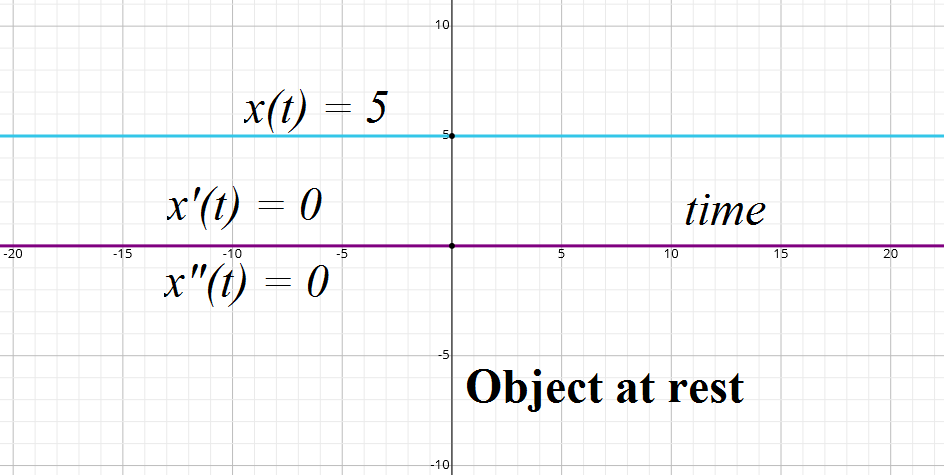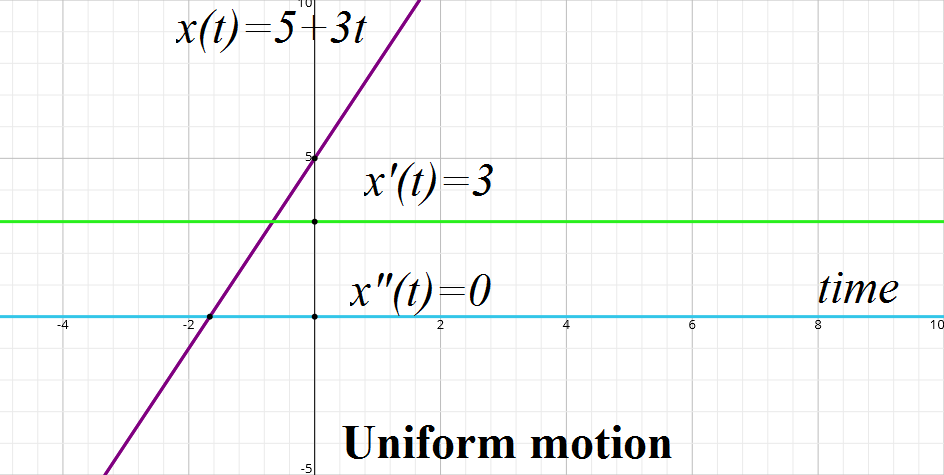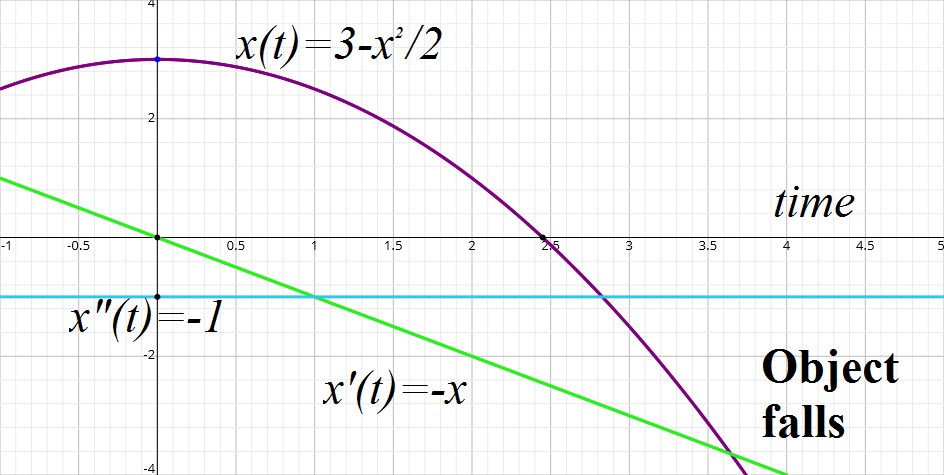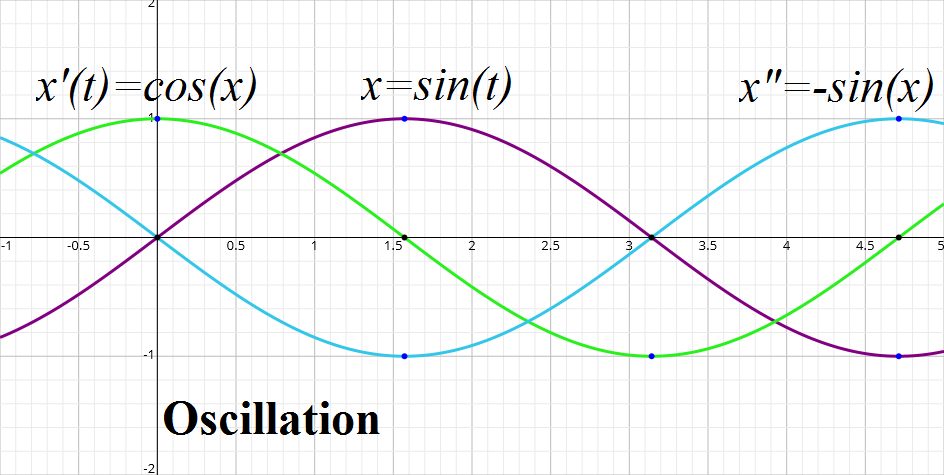## Thursday, April 19, 2018

### Unizor - Physics4Teens - Mechanics - Kinematics

Notes to a video lecture on http://www.unizor.com

Graphical Representation
of Motion

We have studied three categories of functions:

position (coordinate) functions x(t), y(t) and z(t) to define the position of an object in three-dimensional space, using their Cartesian coordinates as functions of time t;

velocity functions x'(t), y'(t) and z'(t) to define the rate of change of position of an object in three-dimensional space; these functions are first derivatives of corresponding position functions;

acceleration functions x"(t), y"(t) and z"(t) to define the rate of change of velocity of an object in three-dimensional space; these functions are second derivatives of corresponding position functions.

In general, to graphically analyze any function y=f(x) of a single argument x, we use two-dimensional plane with XY-coordinates and construct a graph of this function as a set of all points {x, y=f(x)} for all arguments x in the domain of this function.

We will do this analysis for each characteristic of motion as function of time argument t (position, velocity and acceleration) in some simple cases.

Our main case is a motion along the straight line. It simplifies our
analysis, as we can choose an X-axis coinciding with the line of motion,
and Y- and Z-coordinates of an object will always be zero. So, we will
have only one set of functions - x(t), x'(t) and x"(t) to analyze.

We will also extend the time line to both directions, positive (future)
and negative (past), because our null-moment is chosen arbitrarily.

Our first case is an object at rest. Its X-coordinate is constant: x(t)=5. The first and second derivatives of this function are zero: x'(t)=0, x"(t)=0. Graphically, all three characteristics of motion look like this:Next let's consider a uniform motion along an X-axis, according to position function x(t)=5+3t. Its velocity function (the first derivative of position) is x'(t)=3 (constant for uniform motion) and its acceleration (second derivative) is x"(t)=0. Graphically, all three characteristics of motion look like this:Now let's consider a case of an object falling from some height down along an X-axis, according to position function x(t)=3−t²/2. Its velocity function (the first derivative of position) is x'(t)=−t and its acceleration (second derivative) is x"(t)=−1 (constant). Graphically, all three characteristics of motion look like this:Finally, let's consider a case of an object oscillating left and right
along an X-axis around point 0, according to position function x(t)=sin(t). Its velocity function (the first derivative of position) is x'(t)=cos(t) and its acceleration (second derivative) is x"(t)=−sin(t). Graphically, all three characteristics of motion look like this: